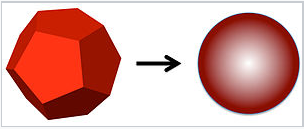# 11.5: Surface Energy

$$\newcommand{\vecs}{\overset { \rightharpoonup} {\mathbf{#1}} }$$ $$\newcommand{\vecd}{\overset{-\!-\!\rightharpoonup}{\vphantom{a}\smash {#1}}}$$$$\newcommand{\id}{\mathrm{id}}$$ $$\newcommand{\Span}{\mathrm{span}}$$ $$\newcommand{\kernel}{\mathrm{null}\,}$$ $$\newcommand{\range}{\mathrm{range}\,}$$ $$\newcommand{\RealPart}{\mathrm{Re}}$$ $$\newcommand{\ImaginaryPart}{\mathrm{Im}}$$ $$\newcommand{\Argument}{\mathrm{Arg}}$$ $$\newcommand{\norm}{\| #1 \|}$$ $$\newcommand{\inner}{\langle #1, #2 \rangle}$$ $$\newcommand{\Span}{\mathrm{span}}$$ $$\newcommand{\id}{\mathrm{id}}$$ $$\newcommand{\Span}{\mathrm{span}}$$ $$\newcommand{\kernel}{\mathrm{null}\,}$$ $$\newcommand{\range}{\mathrm{range}\,}$$ $$\newcommand{\RealPart}{\mathrm{Re}}$$ $$\newcommand{\ImaginaryPart}{\mathrm{Im}}$$ $$\newcommand{\Argument}{\mathrm{Arg}}$$ $$\newcommand{\norm}{\| #1 \|}$$ $$\newcommand{\inner}{\langle #1, #2 \rangle}$$ $$\newcommand{\Span}{\mathrm{span}}$$$$\newcommand{\AA}{\unicode[.8,0]{x212B}}$$

Nanoparticles have a substantial fraction of their atoms on the surface, as shown in the plot at the right. This high surface area to volume ratio is an important factor in many of the physical properties of nanoparticles, such as their melting point and vapor pressure, and also in their reactivity. Heterogeneous catalysts, for example, are often based on nanoparticles because the catalytically reactive atoms are those that are on the surface of the particle.The surface energy is always positive. A key quantity that is connected with the chemistry of all surfaces is the surface energy. This is the (thermodynamically unfavorable) energy of making "dangling bonds" at the surface. Atoms at the surface are under-coordinated, and because breaking bonds costs energy, surface atoms always have higher energy than atoms in the bulk. This happens regardless of whether the bonding is covalent (as in a metal), ionic (in a salt), or non-covalent (in a liquid such as water). We see this effect, for example, in water droplets that bead up on a waxy surface. The droplet contracts into a sphere (against the force of gravity that works to flatten it) in order to minimize the number of dangling hydrogen bonds at the surface.

In the case of metal or semiconductor particles, strong covalent bonds are broken at the surface. For example, a gold atom in bulk face-centered cubic Au has 12 nearest neighbors, but a gold atom on the (111) surface of the crystal (the most dense crystal plane of gold) has six nearest neighbors in-plane and three underneath, for a total of 9. We might expect the surface energy of this crystal face to be a little less (because the remaining bonds will become slightly stronger) than 3/12 = 1/4 of the bonding energy of bulk Au, and this is in fact a fairly good rule of thumb for many materials. When translated into energy per unit area, the surface energy of metals and inorganic salts is usually in the range of 1-2 J/m2.

Example: The sublimation energy of bulk gold is 334 kJ/mol, and the surface energy is 1.5 J/m2. What percentage of the bulk bonding energy is lost by atoms at the (111) surface of a gold crystal?

To solve this problem, we need to know the surface area per Au atom. Gold has the face-centered cubic structure, and the unit cell edge length is 4.08 Å. From this we can determine that the Au-Au distance is 4.08/1.414 = 2.88 Å. In a hexagonal array of gold atoms with this interatomic spacing, the surface area per atom is (2.88 Å)2 x 0.866 = 7.2 Å2. Multiplying by Avogadro's number, we find that the area per mole of Au surface atoms on the (111) crystal face is 4.3 x 104 m2.

Now we multiply this area by the surface energy:

$$(4.3 \times 10^{4} \frac{m^{2}}{mol}) \times (1.5 \frac{J}{mol}) \times (\frac{1kJ}{1000J}) = \mathbf{+65 kJ}$$ per mole of Au surface atoms.

$$\frac{65 kJ}{334kJ} \times 100 \% = \mathbf {19 \%}$$ of the bulk bonding energy is lost by atoms at the surface.

It is clear from this example that the surface energy of nanoparticles can have a major effect on their physical properties, since a large fraction of the atoms in a nanoparticle are on the surface. A good example of this is the dramatic depression of the melting point.

Solid nanocrystals are in general faceted, whereas liquid droplets made by melting nanocrystals adopt a spherical shape to minimize the surface area.A faceted solid nanocrystal melts into a spherical droplet in order to minimize its surface energy.

Let's consider melting a silver nanocrystal that is 2 nm in diameter, meaning that about 1/2 of the atoms are on the surface. The spherical liquid droplet has lower surface area than the faceted crystal. For example, a cube has 1.24 times the surface area of a sphere of the same volume. If the decrease in surface area is about 20% upon melting, and the surface energy is about 1/4 of the bulk bonding energy of the atoms, then for a 2 nm diameter nanocrystal we would estimate that:

$\Delta H^{o}_{fusion} \approx \Delta H^{o}_{fusion, \: bulk} - (\frac{1}{2})(0.25)(\frac{1}{4}) \Delta H^{o}_{vap} = \Delta H^{o}_{fusion, \: bulk} - 0.025 \Delta H^{o}_{vap, \: bulk}$

where ΔH°vap, bulk, the heat of vaporization, is the total bonding energy of atoms in a bulk crystal. For silver, ΔH°fusion, bulk = 11.3 kJ/mol and ΔH°vap, bulk = 250 kJ/mol. From this we can calculate the heat of fusion of a 2 nm Ag nanocrystal:

$\Delta H^{o}_{fusion} \approx 11.3 - (0.025)(25) = 5.1 \frac{kJ}{mol}$

The melting point of bulk silver is 962 °C = 1235 K. Assuming that the entropy of fusion is the same in the bulk and in the nanocrystal, the melting point of the nanocrystal should be $$1234K \times (\frac{5.1}{11.3}) = 557K = 284^{\circ} C$$, a drop of almost 700 degrees from the bulk value. Experimentally, the melting point of a 2 nm diameter silver nanocrystal drops about 800 degrees below that of the bulk, to 127 °C. This is a whopping big change in the melting point, which is in reasonable agreement with our rough estimate.

The same effect - energetic destabilization of the surface atoms relative to bulk atoms - results in the lower boiling point, higher vapor pressure, higher solubility, and higher reactivity of nanocrystals relative to microcrystals (or larger crystals) of the same material.

This page titled 11.5: Surface Energy is shared under a CC BY-SA 4.0 license and was authored, remixed, and/or curated by Chemistry 310 (Wikibook) via source content that was edited to the style and standards of the LibreTexts platform; a detailed edit history is available upon request.